# Generating White Gaussian Noise Using Randn Function in Matlab

Matlab is a great tool for conducting scientific and engineering calculations. This software has a great number of toolboxes that gives a wide variety of possible operations. In the following article we are going to talk about generating white Gaussian noise using Randn function and plot it. If you are just learning how to use Matlab, our guide should help. Besides you can check other samples available on our blog and find more essential information for your engineering classes.

Mathematics, programming, engineering – it’s not a complete list of disciplines that our expert can cover. If you will find out that your discipline is missing in the order form, simply mention it and we will find the expert that will be ready to complete your task. We strive to make the ordering process easy and fast, so it will take no more than five minutes to complete the order form. For your convenience, our service is available seven days a week. Value your time and choose AssignmentShark.com online assignment help.

## Gaussian White Noise Signal

Task: Use Matlab to generate a Gaussian white noise signal of length L=100,000 using the randn function and plot it.

Solution:

Since the random variables in the white noise process are statistically uncorrelated, the covariance function contains values only along the diagonal. The matrix above indicates that only the autocorrelation function exists for each random variable. The cross-correlation values are zero (samples/variables are statistically uncorrelated with respect to each other). The diagonal elements are equal to the variance and all other elements in the matrix are zero.

Plot the histogram of the generated white noise and verify the histogram by plotting against the theoretical pdf of the Gaussian random variable. This can be achieved in a few ways.

Way 1

Code: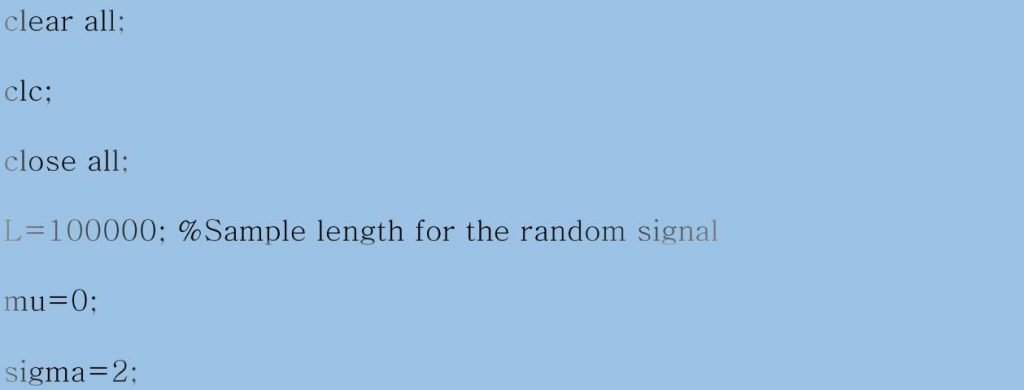Code: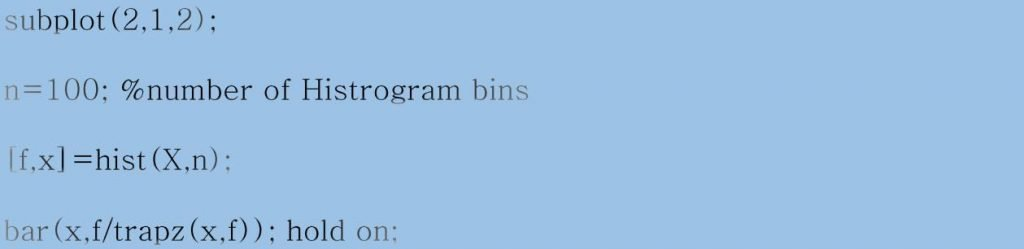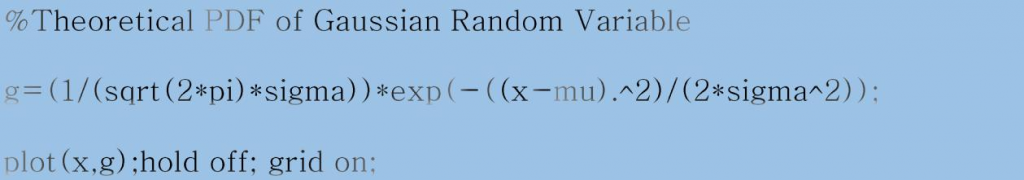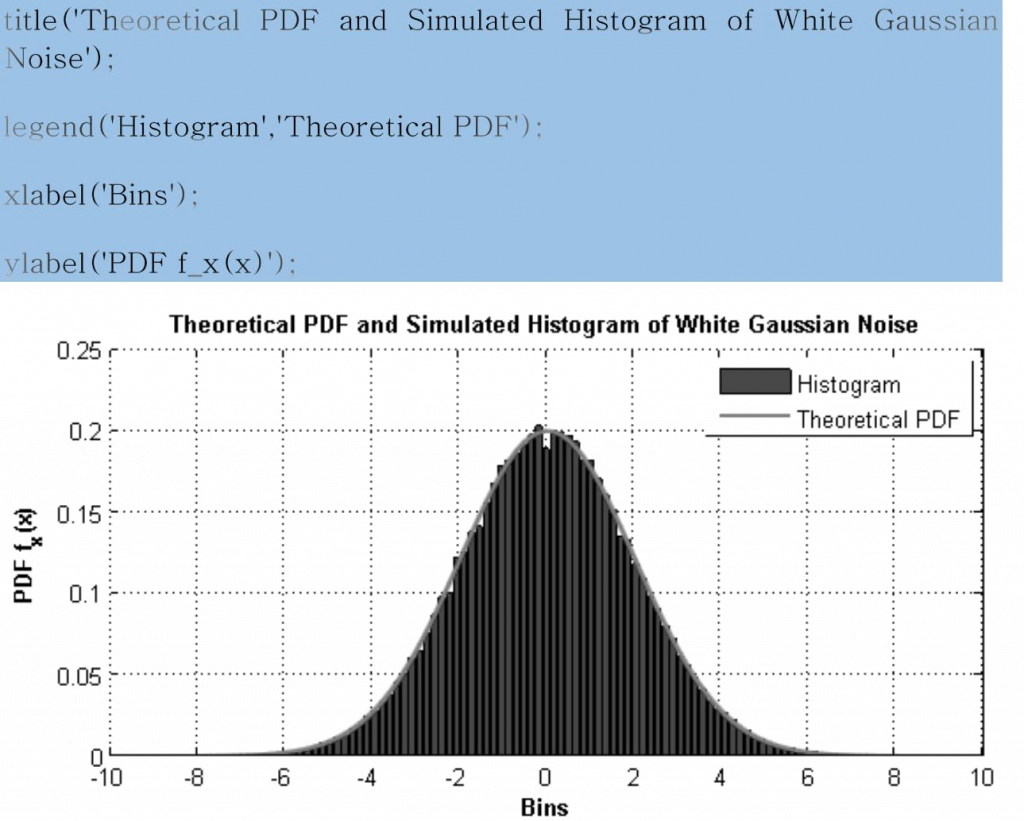The computed autocorrelation function has to be scaled properly. If the ‘xcorr’ function (inbuilt in Matlab) is used for computing the autocorrelation function, use the ‘biased’ argument in the function to scale it properly.

Compute PSD of the above generated multi-dimensional process and average it to get a smooth plot.

Code: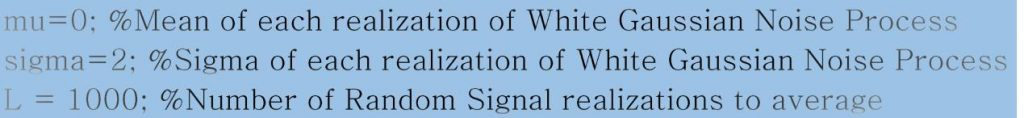The PSD plot of a white noise gives almost fixed power in all the frequencies. In other words, for a white noise signal, the PSD is constant (flat) across all the frequencies (-∞ to + ∞).